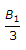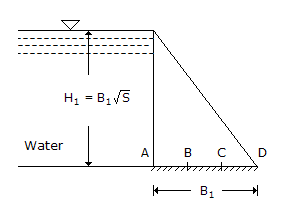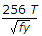# Civil Engineering - UPSC Civil Service Exam Questions

21.

Given figure shows a retaining wall of base width B1 and height H1 The sp. gravity of the material of construction is S. Further AB = BC = CD =When the depth of storage increases from 0 to H1, the resultant force will move fromA. A to B B. B to C C. C to D D. B to D

Explanation:

No answer description available for this question. Let us discuss.

22.

A machine costs Rs. 16, 000. By constant rate of declining balance method of depreciation, its salvage value after an expected life of 3 years is Rs. 2, 000. The rate of depreciation is

 A. 0.25 B. 0.3 C. 0.4 D. 0.5

Explanation:

No answer description available for this question. Let us discuss.

23.

Consider the following statements regarding the longitude of a place.
It is determined by :
1. wireless time signals
2. transportation of chronometers
3. electric telegraph.
Of these statements :

 A. 1, 2 and 3 are correct B. 1 and 2 are correct C. 1 and 3 are correct D. 2 and 3 are correct

Explanation:

No answer description available for this question. Let us discuss.

24.

The outstand of the flange of built-up beams from the line of connection should not extend beyond:(where T is the thickness of flange and tw is the thickness of web)

 A. 10 T B. 85 T C.D. 180tw

Explanation:

No answer description available for this question. Let us discuss.

25.

Consider the following statements :
In subsoil exploration programme the term "significant depth of exploration" is upto
1. the width of foundation
2. twice the width of foundation
3. the depth where the additional stress intensity is less than 20% of overburden pressure
4. the depth where the additional stress intensity is less than 10% of the overburden pressure
5. hard rock level.
Of these statements :

 A. 1, 3 are 5 are correct B. 2, 3 and 5 are correct C. 1 and 4 are correct D. 2 and 4 are correct